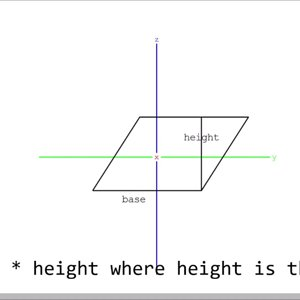# Rectangle Definition and 6 Discussions

In Euclidean plane geometry, a rectangle is a quadrilateral with four right angles. It can also be defined as: an equiangular quadrilateral, since equiangular means that all of its angles are equal (360°/4 = 90°); or a parallelogram containing a right angle. A rectangle with four sides of equal length is a square. The term oblong is occasionally used to refer to a non-square rectangle. A rectangle with vertices ABCD would be denoted as ABCD.
The word rectangle comes from the Latin rectangulus, which is a combination of rectus (as an adjective, right, proper) and angulus (angle).
A crossed rectangle is a crossed (self-intersecting) quadrilateral which consists of two opposite sides of a rectangle along with the two diagonals (therefore only two sides are parallel). It is a special case of an antiparallelogram, and its angles are not right angles and not all equal, though opposite angles are equal. Other geometries, such as spherical, elliptic, and hyperbolic, have so-called rectangles with opposite sides equal in length and equal angles that are not right angles.
Rectangles are involved in many tiling problems, such as tiling the plane by rectangles or tiling a rectangle by polygons.

View More On Wikipedia.org
1. ### Buoyancy with the Cross-Sectional Area of a Rectangle

Hi all, My teacher assigned us a problem to do a few days ago and have attempted it many times, often leaving and coming back to see if I could figure it out. I imagine that you would take the cross-sectional area and multiply it by how far under the surface of the water the rectangular object...
2.### Parallelogram Area Formula Origin - YouTube

This is my first video!
3. ### I Möbius strip question

How can the edge of a Möbius strip being projected on a 2 dimensional plane? Precisely the ending of this video: I just can get it since his animation goes by it so fast.
4. ### B Stumped by the simplest geometry problem

Yes, one more reason to be humble, I know. This is the simplest problem I couldn't solve so far. Assume we have a circle of center O, a ruler of arbitrary size and a pencil. We use the ruler and the pencil to choose 4 points on the circle - the extremities of two diametral/diagonal segments...
5. ### Minimum of x+1/x (Perimeter Square < Perim equal area rects)

Homework Statement Gelfand - Algebra p.115 problem 264: Prove that a square has the minimum perimeter of all rectangles having the same area. Hint. Use the result of the preceding problem. Homework Equations Preceding problem: Prove that a square has the maximum area of all rectangles having...
6. ### Permutations and combinations - is square a rectangle?

I was going through a p and c problem where I had to find the number of non congruent RECTANGLES. Answer includes number of squares as well. SHOULD SQUARE BE TAKEN AS A RECTANGLE?